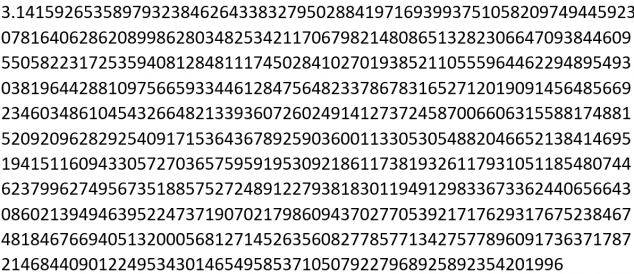# Pi Day – William Shanks, the human calculator who made a mistake

It’s time to celebrate Pi Day again – Happy 14th March! (Or 3.14 in the U.S. where the month and date are written the other way around.) This year we will look at the life and work of William Shanks, an amateur mathematician who devoted his life to calculating the digits of Pi, only to make a mistake part way through.

Pi is an irrational number – this means that the decimal expansion goes on forever, without repeating any kind of regular pattern.  People have been calculating Pi for thousands of years – with Archimedes having calculated 2 decimal places by 250 BC. By the time that William Shanks was born in 1812, the world record was a calculation of Pi correct to 152 decimal places.

### Do we need lots of digits of Pi?

Engineers, scientists and architects need to use Pi all the time, however they don’t actually need many decimal places in order to make their calculations. Even NASA say that they don’t need Pi to be accurate to more than 16 decimal places. Calculating lots of digits of Pi doesn’t have a lot of practical uses, however it has still captured the imaginations of countless enthusiasts.

Before computers were invented, digits of Pi had to be calculated by hand, making each new digit a labour of love which took many days or months to complete. As soon as computers came on the scene, it was much easier to calculate the digits of Pi – the challenge was now more about building the biggest and fastest computer. The most recent record was created on Pi Day in 2019 by Google, who calculated Pi to 31.4 trillion decimal places!

### Who was William Shanks?

William Shanks was born in 1812 near Durham in the North East of England. He ran a small boarding school, but spent a lot of his spare time calculating the digits of Pi. He would work all morning on his calculations, before spending the afternoon checking over his work. (How he had time to run a boarding school is anybody’s guess!) William Shanks didn’t limit himself to calculating the digits of Pi – he also calculated the digits of the mathematical constants$e$ and$\gamma$, as well as making a list of the first 60,000 prime numbers, and calculating natural logarithms with bases 2,3,5 and 10. In his day William Shanks was known as a ‘computer’, he was a human version of the modern day electronic computer.

### How did Shanks calculate Pi?

William Shanks used Machin’s formula in order to calculate the digits of Pi.

Machin’s Formula:$\large \frac{\pi}{4}=4 \tan ^{-1}\left(\frac{1}{5}\right)-\tan ^{-1}\left(\frac{1}{239}\right)$

A Taylor series can then be used to calculate$\tan ^{-1}(x)$ which is also known as$\arctan (x)$:$\large \tan ^{-1}(x)=\arctan (x)=x-\frac{x^{3}}{3}+\frac{x^{5}}{5}-\frac{x^{7}}{7}+\cdots$

This is an infinite expansion, which means that it goes on forever, and as you calculate more terms, you will get a more and more accurate value.

### The Big Mistake

By 1873 Shanks had calculated Pi to a whopping 707 decimal places. This remained unchallenged until 1944 when D F Ferguson found that Shanks had made a mistake in calculating two of his terms, meaning that his calculation for Pi was only correct for the first 527 digits. Fortunately, Shanks didn’t find out in his lifetime about his mistake, and he has still gone down in history as one of the greatest Pi hunters who has ever lived. In some ways, Shanks’ big mistake has only added to his fame.

We can only guess what could have motivated a man like Shanks, and what drove him to devote most of his life to calculating the digits of Pi. Now we can wipe away his mistake in a few seconds by googling the first 707 digits of Pi. Happy Pi Day!Article by Hazel Lewis